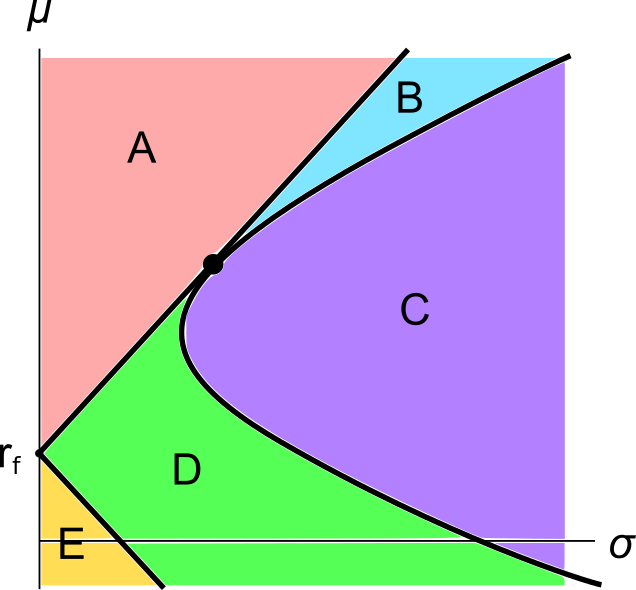# Fight Finance

#### CoursesTagsRandomAllRecentScores

 Portfolio Details Stock Expected return Standard deviation Correlation Dollars invested A 0.1 0.4 0.5 60 B 0.2 0.6 140

What is the expected return of the above portfolio?

One year ago you bought $100,000 of shares partly funded using a margin loan. The margin loan size was$70,000 and the other \$30,000 was your own wealth or 'equity' in the share assets.

The interest rate on the margin loan was 7.84% pa.

Over the year, the shares produced a dividend yield of 4% pa and a capital gain of 5% pa.

What was the total return on your wealth? Ignore taxes, assume that all cash flows (interest payments and dividends) were paid and received at the end of the year, and all rates above are effective annual rates.

Hint: Remember that wealth in this context is your equity (E) in the house asset (V = D+E) which is funded by the loan (D) and your deposit or equity (E).

An investor wants to make a portfolio of two stocks A and B with a target expected portfolio return of 12% pa.

• Stock A has an expected return of 10% pa and a standard deviation of 20% pa.
• Stock B has an expected return of 15% pa and a standard deviation of 30% pa.

The correlation coefficient between stock A and B's expected returns is 70%.

What will be the annual standard deviation of the portfolio with this 12% pa target return?

An investor wants to make a portfolio of two stocks A and B with a target expected portfolio return of 6% pa.

• Stock A has an expected return of 5% pa.
• Stock B has an expected return of 10% pa.

What portfolio weights should the investor have in stocks A and B respectively?

An investor wants to make a portfolio of two stocks A and B with a target expected portfolio return of 16% pa.

• Stock A has an expected return of 8% pa.
• Stock B has an expected return of 12% pa.

What portfolio weights should the investor have in stocks A and B respectively?

An investor owns a portfolio with:

• 80% invested in stock A; and
• 20% invested in stock B.

Today there was a:

• 10% rise in stock A's price; and
• No change in stock B's price.

No dividends were paid on either stock. What was the total historical portfolio return on this day? All returns above and answer options below are given as effective daily rates.

Which of the following assets would you expect to have the highest required rate of return? All values are current market values.

A graph of assets’ expected returns $(\mu)$ versus standard deviations $(\sigma)$ is given in the below diagram.

Each letter corresponds to a separate coloured area. The portfolios at the boundary of the areas, on the black lines, are excluded from each area. Assume that all assets represented in this graph are fairly priced, and that all risky assets can be short-sold.